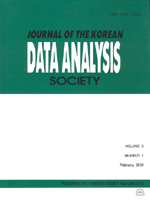상세검색
최근 검색어 전체 삭제
다국어입력
즐겨찾기0KCI등재 학술저널

# Probabilities of overfitting of Model Selection Criteria in Regression

• 등재여부 : KCI등재
• 2001.09
• 245 - 253 (9 pages)

In this paper, we derived overfitted probabilities for model selection criteria in orthogonal regression for two different situations. First, there is one candidate model with one extra variable is compared to the current model. Second, we expanded to m candidate models. We assume that these comparisons are independent and discuss overfitting probabilities. We found the overfitting probabilities of AIC, AICc, SIC, SICc, and HQ on one extra variable case and multiple extra variable case.

1. Introduction

2. Orthogonal regression model

3. Review of model selection criteria

4. Probabilities of overfitting

5. Conclusion and Further Research

References